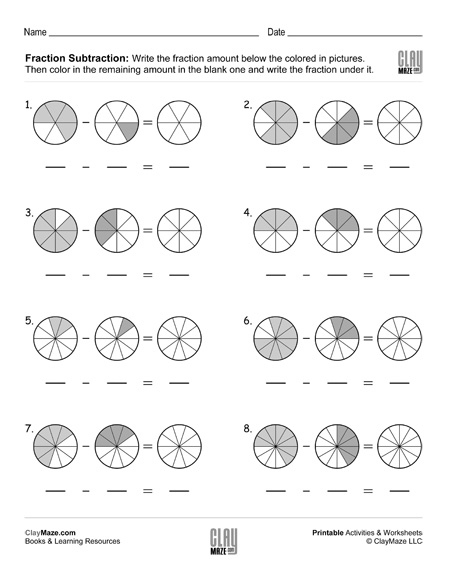Fraction Subtraction with Same Denominators (Set 4)

Fraction subtraction with common denominators worksheet – Write the correct fraction amount under each picture. Subtract the fractions and color the fractional amount in the blank picture.